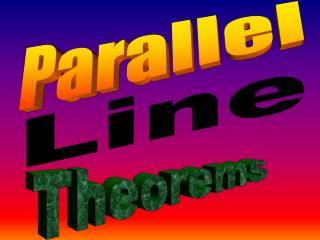DownloadDownload PresentationTheorems

# Theorems

Download Presentation## Theorems

- - - - - - - - - - - - - - - - - - - - - - - - - - - E N D - - - - - - - - - - - - - - - - - - - - - - - - - - -
##### Presentation Transcript

1. Parallel Line Theorems

2. Parallel lines are like train tracks.They never cross.

3. Parallel Line Theorems Two parallel lines on their own are not vary interesting to us in terms of geometry. But if straight line (called a transversal) intersects two parallel lines, then the angles created are very interesting to us…..

4. These lines are parallel because they will never cross…. The dashed line is called a transversal, because it crosses both lines

5. As soon as the transversal is drawn, a collection of angles are created. These angles are equal because of OAT. Copy these to your sheet. x y x y x x y y In this diagram, there is a collection of patterns that can be generated.

6. y y “Z” pattern (alternate angles: AA)

7. x x “Z” pattern

8. y x x y “F” pattern (corresponding angles: CA)

9. x y x y “F” pattern

10. y x X + Y = 180O x y “C” pattern (supplementary angles: SA) ( also called interior angles )

11. Find X,Y and Z Statement Reason 70O Z Z = 70O OAT Y = 180O – 70O SA = 110O Y X X = 110O OAT

12. HOMEWORKsee sheet